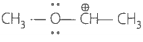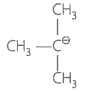The correct order of decreasing stability of the following cations is:

 I $$~~~~~~~~~~~~\oplus\\ CH_3 - CH-CH_3$$ II $$~~~~~~~~~~~~\oplus\\ CH_3 - CH-OCH_3$$ III $$~~~~~~~~~~~~\oplus\\ CH_3 - CH-CH_2- OCH_3$$

 1 II>I>III 2 I>II>III 3 IISubtopic:  Electron Displacement Effects |
57%
From NCERT
To view explanation, please take trial in the course.
NEET 2023 - Target Batch - Aryan Raj Singh
Hints
To view explanation, please take trial in the course.
NEET 2023 - Target Batch - Aryan Raj Singh

Which of the following carbon marked with asterisk is expected to have greatest positive charge?

1. $*{\mathrm{CH}}_{3}-{\mathrm{CH}}_{2}-\mathrm{Cl}$

2. $*{\mathrm{CH}}_{3}-{\mathrm{CH}}_{2}-{\mathrm{Mg}}^{+}{\mathrm{Cl}}^{-}$

3. $*{\mathrm{CH}}_{3}-{\mathrm{CH}}_{2}-\mathrm{Br}$

4. $*{\mathrm{CH}}_{3}-{\mathrm{CH}}_{2}-{\mathrm{CH}}_{3}$Subtopic:  Electron Displacement Effects |
58%
From NCERT
To view explanation, please take trial in the course.
NEET 2023 - Target Batch - Aryan Raj Singh
Hints
To view explanation, please take trial in the course.
NEET 2023 - Target Batch - Aryan Raj Singh

The most stable carboxylate ion among the following is-

1. ${\mathrm{CH}}_{3}-\stackrel{\mathrm{O}}{\stackrel{||}{\mathrm{C}}}-{\mathrm{O}}^{-}$

2. $\mathrm{Cl}-{\mathrm{CH}}_{2}-\stackrel{\mathrm{O}}{\stackrel{||}{\mathrm{C}}}-{\mathrm{O}}^{-}$

3. $\mathrm{F}-{\mathrm{CH}}_{2}-\stackrel{\mathrm{O}}{\stackrel{||}{\mathrm{C}}}-{\mathrm{O}}^{-}$

4. ${\left(\mathrm{F}\right)}_{2}-\mathrm{CH}-\stackrel{\mathrm{O}}{\stackrel{||}{\mathrm{C}}}-{\mathrm{O}}^{-}$Subtopic:  Electron Displacement Effects |
78%
From NCERT
To view explanation, please take trial in the course.
NEET 2023 - Target Batch - Aryan Raj Singh
Hints
To view explanation, please take trial in the course.
NEET 2023 - Target Batch - Aryan Raj Singh

Match the ions given in Column I with their nature-given in Column II.

 Column I Column II A.1. Stable due to resonance B. ${\mathrm{F}}_{3}-{\mathrm{C}}^{+}$ 2. Destabilised due to inductive effect C.3. Stabilised by hyperconjugation. D. ${\mathrm{CH}}_{3}-\stackrel{\oplus }{\mathrm{C}}\mathrm{H}-{\mathrm{CH}}_{3}$ 4. A secondary carbocation

Codes

 Options: A B C D 1. 1,2 2 2 3,4 2. 1 2,4 3 4 3. 1 4 3 2 4. 4 1 3 2Subtopic:  Electron Displacement Effects | Reaction Intermediates ; Preparation & Properties |
75%
From NCERT
To view explanation, please take trial in the course.
NEET 2023 - Target Batch - Aryan Raj Singh
Hints
To view explanation, please take trial in the course.
NEET 2023 - Target Batch - Aryan Raj Singh

Hyperconjugation involves delocalization of -

 a. Electrons of carbon-hydrogen $\mathrm{\sigma }$ bond of an alkyl group directly attached to an atom of unsaturated system. b. Electrons of carbon-hydrogen $\mathrm{\sigma }$ bond of alkyl group directly attached to the positively charged carbon atom. c. $\mathrm{\pi }$-electrons of carbon-carbon bond d. Lone pair of electrons.

Choose the correct option :

1. (a, b)

2. (b, c)

3. (c, d)

4. (a, c)Subtopic:  Electron Displacement Effects |
67%
From NCERT
To view explanation, please take trial in the course.
NEET 2023 - Target Batch - Aryan Raj Singh
Hints
To view explanation, please take trial in the course.
NEET 2023 - Target Batch - Aryan Raj Singh